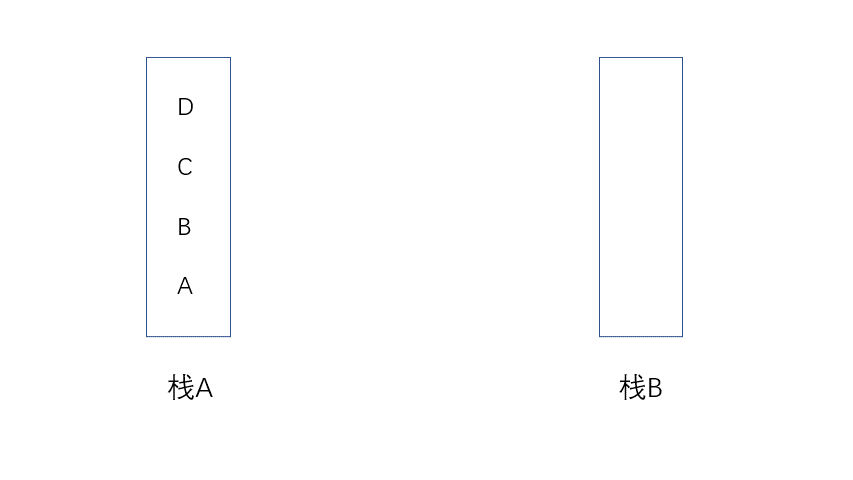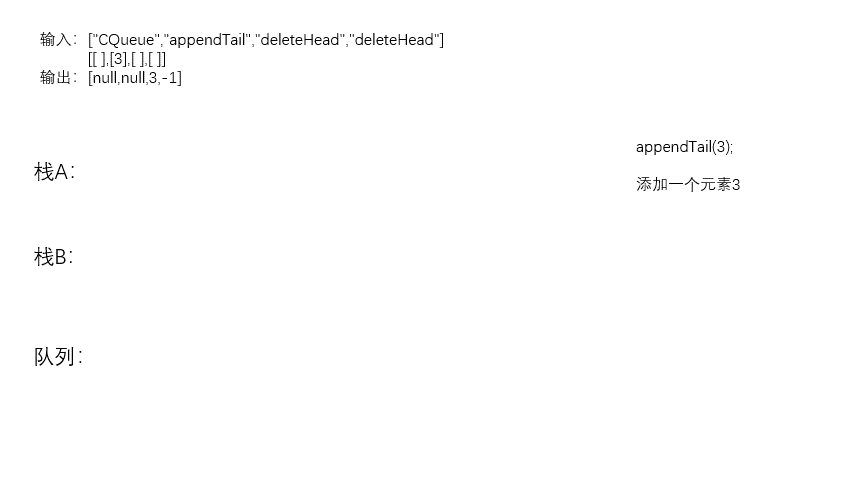# Queue with two stacks

2020-11-08 21:03:45

# Queues are implemented with two stacks

## subject

Use two stacks to implement a queue . The declaration of the queue is as follows , Please implement its two functions appendTail and deleteHead , The functions of inserting integers at the end of the queue and deleting integers at the head of the queue are respectively completed .( If there are no elements in the queue ,deleteHead  Operation return -1 )

`````` Example 1 ：
Input ：
[[],,[],[]]
Output ：[null,null,3,-1]

Example  2：
Input ：
[[],[],,,[],[]]
Output ：[null,-1,null,null,5,2]
``````

Tips ：

• 1 <= values <= 10000
• At most appendTail、deleteHead Conduct 10000 Secondary call

source ： Power button （LeetCode）

## Their thinking

The topic requires stack implementation , Complete the function of inserting integers at the end of the queue and deleting integers at the head of the queue .

The characteristic of stack is first in first out , The elements that are first put on the stack are finally put out of the stack , If you want to delete the element at the bottom of the stack , Other elements can only be pushed out of the stack .

At this point, you can use two stacks , Stack A Elements come out of the stack and into B in , This is on the stack A The element at the bottom of the stack is the stack B Top element of . Now the stack B Out of the stack is equivalent to deleting the stack A At the bottom of the stack .So when it comes to completing the function , You can use stacks A Implement the integer insertion at the end of the queue , Utilization stack B Reverse the stack A, Stack B The top element of the stack is put out of the stack to delete the integer of the queue head .

Now the stack B There are several situations when the top element of the stack is released ：

• Stack A Not empty , Stack B It's empty time , Stack A The element goes to the stack B in , Back to the stack B Top element of , The stack A At the bottom of the stack
• Stack A It's empty , Stack B Also empty , Neither stack has elements , Indicates that there are no elements in this queue ,deleteHead Operation return -1
• Stack B Isn't empty , Now the stack B There are still inverted elements in , Stack B The top of the stack is still a stack A At the bottom of the stack （ Because the stack is first in and then out , Even if the stack A Added elements or stacks A There are still elements in it , But it doesn't affect the stack B The top element of the stack is the stack A At the bottom of the stack ）
`````` Example 1 ：

Input ：

[[],,[],[]]

Output ：[null,null,3,-1]
``````author ：jyd
source ： Power button （LeetCode）

## Code implementation

``````class CQueue {

public CQueue() {
// Create a stack A And the stack B And initialization
}

public void appendTail(int value) {
}

// There are three situations

//1.  Stack A,B It's all empty time , Indicates that the queue has no data
if(A.isEmpty()&& B.isEmpty()){
return -1;
}

//2.  When B Isn't empty , Output B Top element of
if(!B.isEmpty()){
return B.removeLast();
}

//3.  When A Not empty ,B It's empty time , To put A Data in go to B in
while(!A.isEmpty()){
}
return B.removeLast();
}
}
``````